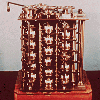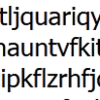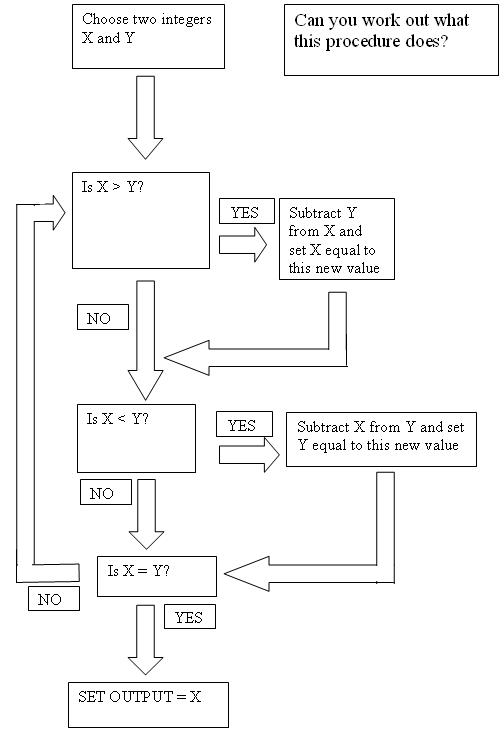#### You may also like### Route to Root

A sequence of numbers x1, x2, x3, ... starts with x1 = 2, and, if you know any term xn, you can find the next term xn+1 using the formula: xn+1 = (xn + 3/xn)/2 . Calculate the first six terms of this sequence. What do you notice? Calculate a few more terms and find the squares of the terms. Can you prove that the special property you notice about this sequence will apply to all the later terms of the sequence? Write down a formula to give an approximation to the cube root of a number and test it for the cube root of 3 and the cube root of 8. How many terms of the sequence do you have to take before you get the cube root of 8 correct to as many decimal places as your calculator will give? What happens when you try this method for fourth roots or fifth roots etc.?### Divided Differences

When in 1821 Charles Babbage invented the `Difference Engine' it was intended to take over the work of making mathematical tables by the techniques described in this article.### Probably a Code?

Is the regularity shown in this encoded message noise or structure?

# Procedure Solver

##### Age 16 to 18Challenge LevelCan you prove that the algorithm will always work?

NOTES AND BACKGROUND

When computers do their various jobs they follow very clearly described procedures. These are series of instructions where the next step at each stage is 100% clearly described: no choices or decisions need to be made; the computer is told exactly what to do. The art of the computer programmer is to work out how to break down a task into such a series of steps so that the computer knows exactly how to perform that task.

The procedure in this question is an example of such a breakdown. The computer user would input the two numbers X and Y and the computer would then run through the procedure until it terminates.

Thinking in this procedural way is an important skill to develop for anyone wishing to learn how to program a computer (and perhaps to earn a great deal of money!).